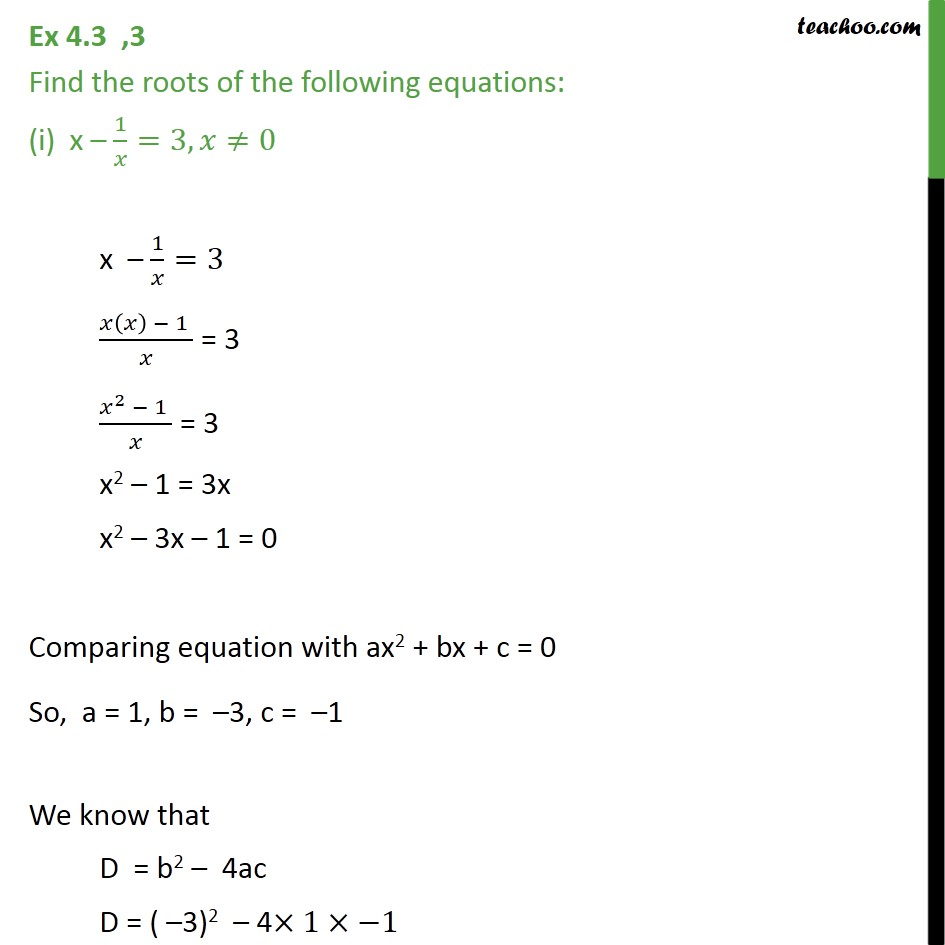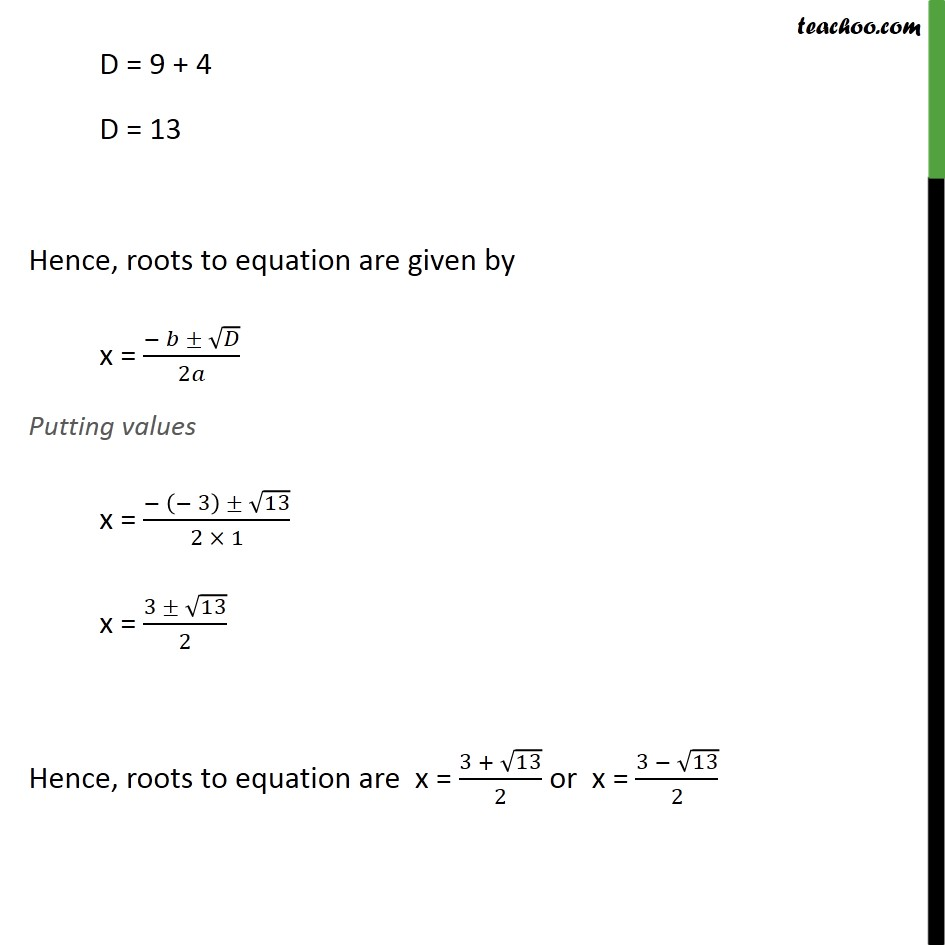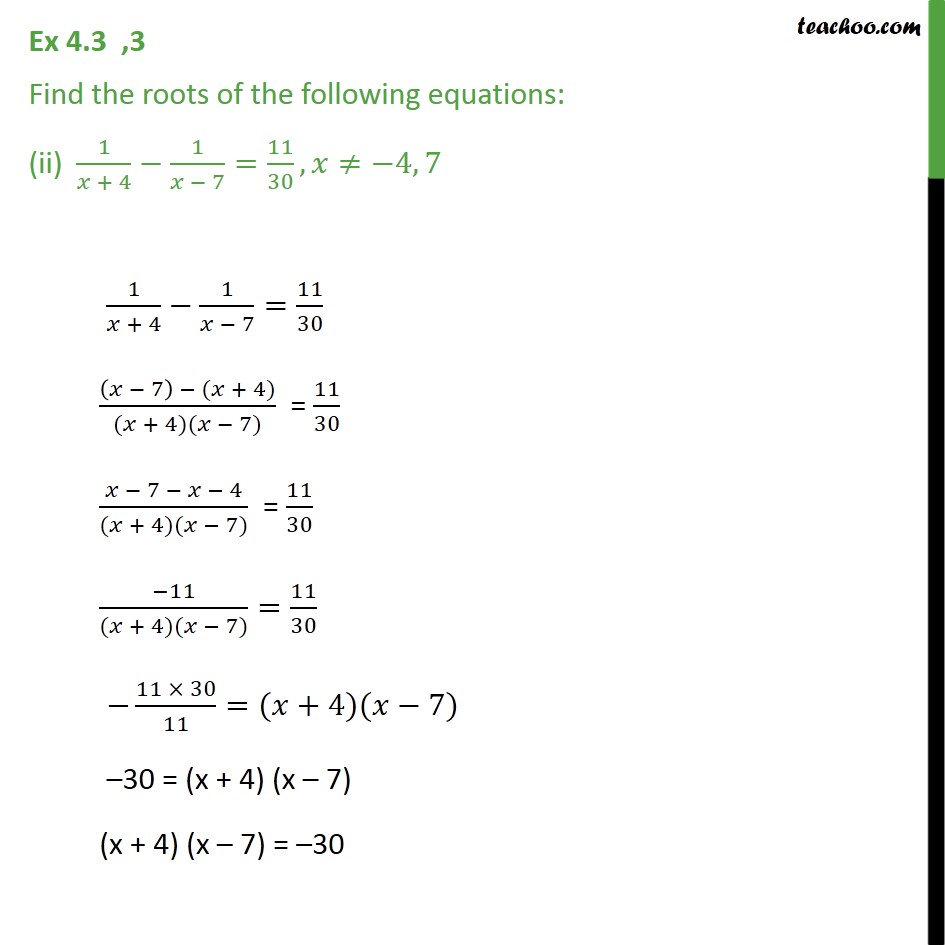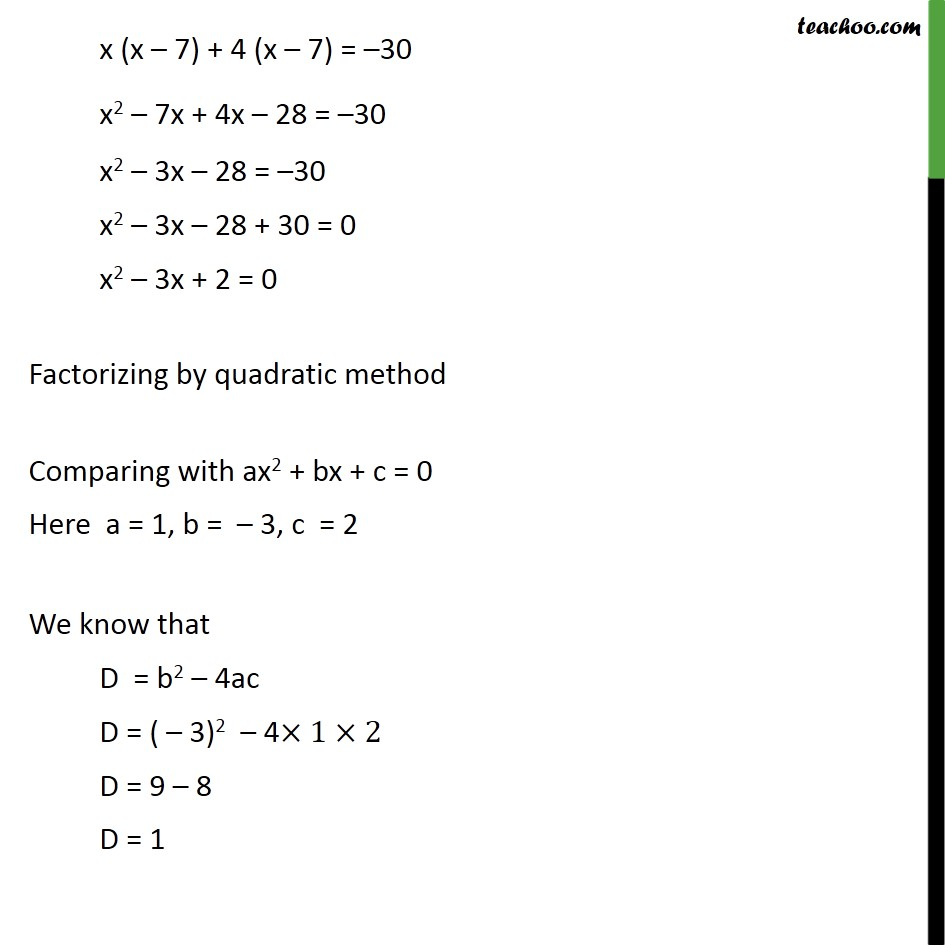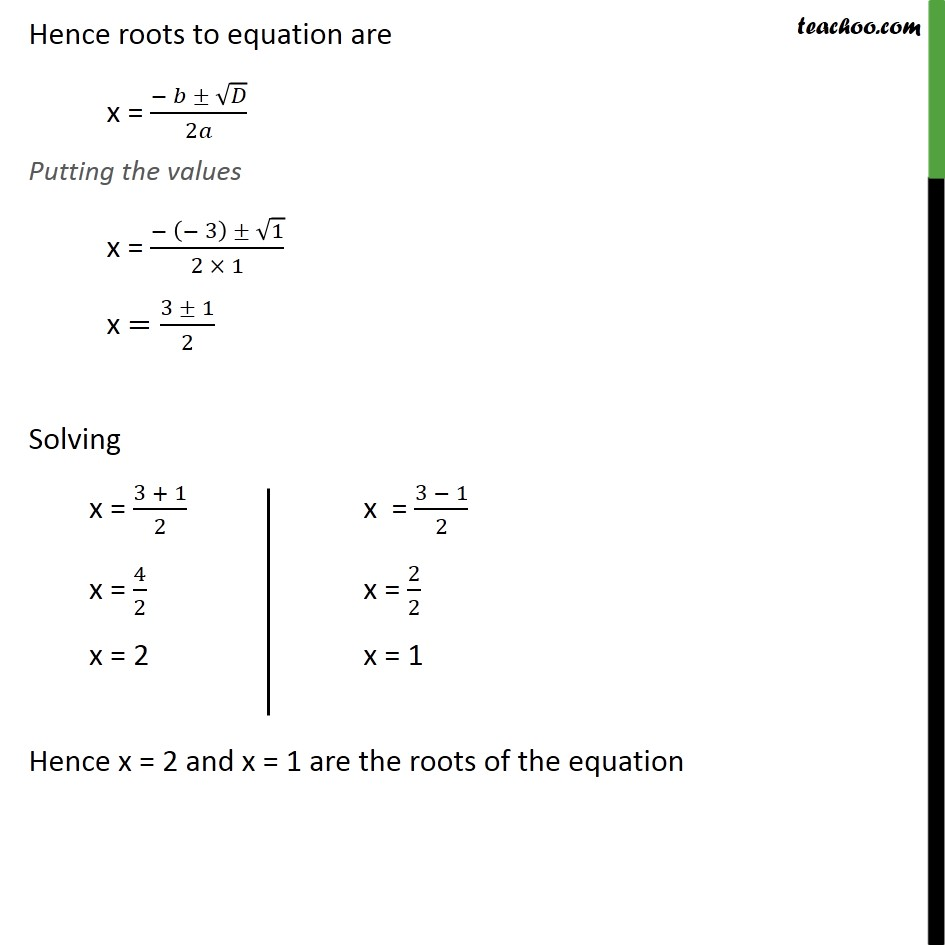Subscribe to our Youtube Channel - https://you.tube/teachoo

1. Chapter 4 Class 10 Quadratic Equations
2. Serial order wise
3. Ex 4.3

Transcript

Ex 4.3 ,3 Find the roots of the following equations: (i) x – 1/𝑥=3,𝑥≠0 x – 1/𝑥=3 (𝑥(𝑥) − 1 )/𝑥 = 3 (𝑥^2 − 1 )/𝑥 = 3 x2 – 1 = 3x x2 – 3x – 1 = 0 Comparing equation with ax2 + bx + c = 0 So, a = 1, b = –3, c = –1 We know that D = b2 – 4ac D = ( –3)2 – 4×1×−1 D = 9 + 4 D = 13 Hence, roots to equation are given by x = (− 𝑏 ± √𝐷)/2𝑎 Putting values x = (− (− 3) ± √13)/(2 × 1) x = (3 ± √13)/2 Hence, roots to equation are x = (3 + √13)/2 or x = (3 − √13)/2 Ex 4.3 ,3 Find the roots of the following equations: (ii) 1/(𝑥 + 4)−1/(𝑥 − 7)=11/30,𝑥≠−4, 7 1/(𝑥 + 4)−1/(𝑥 − 7)=11/30 ((𝑥 − 7) − (𝑥 + 4))/((𝑥 + 4)(𝑥 − 7)) = 11/30 (𝑥 − 7 − 𝑥 − 4)/((𝑥 + 4)(𝑥 − 7)) = 11/30 (−11)/((𝑥 + 4)(𝑥 − 7))=11/30 −(11 × 30)/11=(𝑥+4)(𝑥−7) –30 = (x + 4) (x – 7) (x + 4) (x – 7) = –30 x (x – 7) + 4 (x – 7) = –30 x2 – 7x + 4x – 28 = –30 x2 – 3x – 28 = –30 x2 – 3x – 28 + 30 = 0 x2 – 3x + 2 = 0 Factorizing by quadratic method Comparing with ax2 + bx + c = 0 Here a = 1, b = – 3, c = 2 We know that D = b2 – 4ac D = ( – 3)2 – 4×1×2 D = 9 – 8 D = 1 Hence roots to equation are x = (− 𝑏 ± √𝐷)/2𝑎 Putting the values x = (− (− 3) ± √1)/(2 × 1) x =(3 ± 1)/2 Solving Hence x = 2 and x = 1 are the roots of the equation

Ex 4.3